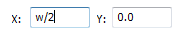# Calculation Edit BoxesNumerical edit boxes generally support simple calculations.

A sum can be entered directly into the edit box:Having typed an equation, pressing the = key will perform the entered calculation and fill the edit box with the answer.

## Special Calculation Characters

As well as the simple numerical calculations, such as 3+(4/5), several of Cut2D Desktop's stored values can be accessed by using certain letters (which are not case-sensitive): When used, Cut2D Desktop substitutes the character with the appropriate value in the calculation.

Character

Name

Example

Description

W or X

Material Width

`w/2=`

Half of the material width

H or Y

Material Height

`H*2=`

Twice the height of the material

T or Z

Material Thickness

`t-0.25=`

0.25 units less than the material thickness

P

PI (3.141593)

`P*10^2=`

Area of a 10 radius circle (π.r2)

I

Imperial Conversion

`25.4*i=`

Converts 25.4mm to inches

M

Metric Conversion

`2*M=`

Converts 2 inches to millimetres

'

Feet

`2'+10=`

34 inches (2 feet and 10 inches)